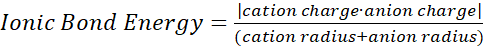# Problem: Which compound in each of the following pairs has the larger lattice energy? Note: Mg2+ and Li+ have similar radii; O2– and F– have similar radii. Explain your choices.(b) LiF or MgO

###### FREE Expert Solution

•  Lattice Energy is the energy required to combine gaseous ions into a solid, crystalline structure.

•  Lattice Energy can be approximated using the equation for ionic bond energy

The higher the ionic bond energy, the greater the lattice energywhere radius is the period number

97% (159 ratings)###### Problem Details

Which compound in each of the following pairs has the larger lattice energy? Note: Mg2+ and Li+ have similar radii; O2– and F have similar radii. Explain your choices.
(b) LiF or MgO